# How to count words in Excel

The tutorial explains how to count words in Excel by using the LEN function in combination with other Excel functions, and provides case-sensitive and case-insensitive formulas to count total or specific words/text in a cell or range.

Microsoft Excel has a handful of useful functions that can count nearly everything: the COUNT function to count cells with numbers, COUNTA to count non-blank cells, COUNTIF and COUNTIFS to conditionally count cells, and LEN to calculate the length of a text string.

Unfortunately, Excel doesn't provide any built-in tool for counting the number of words. Luckily, by combining serval functions you can make more complex formulas to accomplish almost any task. And we will be using this approach to count words in Excel.

## How to count the total number of words in a cell

To count words in a cell, use the following combination of LEN, SUBSTITUTE and TRIM functions:

LEN(TRIM(cell))-LEN(SUBSTITUTE(cell," ",""))+1

Where cell is the address of the cell where you want to count words.

For example, to count words in cell A2, use this formula:

`=LEN(TRIM(A2))-LEN(SUBSTITUTE(A2," ",""))+1`

And then, you can copy the formula down to count words in other cells of column A: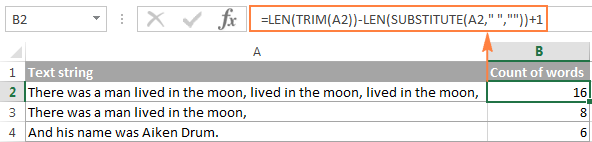#### How this word counting formula works

First, you use the SUBSTITUTE function to remove all spaces in the cell by replacing them with an empty text string ("") for the LEN function to return the length of the string without spaces:

`LEN(SUBSTITUTE(A2," ",""))`

After that, you subtract the string length without spaces from the total length of the string, and add 1 to the final word count, since the number of words in a cell equals to the number of spaces plus 1.

Additionally, you use the TRIM function to eliminate extra spaces in the cell, if any. Sometimes a worksheet may contain a lot of invisible spaces, for example two or more spaces between words, or space characters accidentally typed at the beginning or end of the text (i.e. leading and trailing spaces). And all those extra spaces can throw your word count off. To guard against this, before calculating the total length of the string, we use the TRIM function to remove all excess spaces except for single spaces between words.

#### Improved formula that properly handles empty cells

The above formula to count words in Excel could be called perfect if not for one drawback - it returns 1 for empty cells. To fix this, you can add an IF statement to check for blank cells:

`=IF(A2="", 0, LEN(TRIM(A2))-LEN(SUBSTITUTE(A2," ",""))+1)`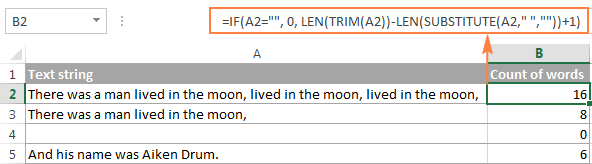As you can see in the screenshot above, the formula returns zero for blank cells, and the correct word count for non-empty cells.

## How to count specific words in a cell

To count how many times a certain word, text, or substring appears in a cell, use the following formula:

`=(LEN(cell)-LEN(SUBSTITUTE(cell, word,"")))/LEN(word)`

For example, let's calculate the number of "moon" occurrences in cell A2:

`=(LEN(A2)-LEN(SUBSTITUTE(A2, "moon","")))/LEN("moon")`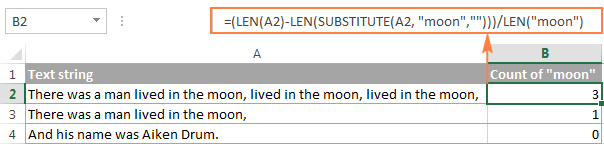Instead of entering the word to be counted directly in the formula, you can type it in some cell, and reference that cell in your formula. As a result, you will get a more versatile formula to count words in Excel.

Tip. If you plan on copying your formula to multiple cells, be sure to fix the reference to the cell containing the word to count with the \$ sign. For example:
`=(LEN(A2)-LEN(SUBSTITUTE(A2, \$B\$1,"")))/LEN(\$B\$1)`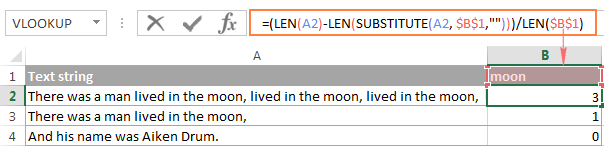#### How this formula counts the occurrences of a specific text in a cell

1. The SUBSTITUTE function removes the specified word from the original text.
In this example, we remove the word input in cell B1 from the original text located in A2:
`SUBSTITUTE(A2, \$B\$1,"")`
2. Then, the LEN function calculates the length of the text string without the specified word.
In this example, `LEN(SUBSTITUTE(A2, \$B\$1,""))` returns the length of the text in cell A2 after removing all characters contained in all occurrences of the word "moon".
3. After that, the above number is subtracted from the total length of the original text string:
`(LEN(A2)-LEN(SUBSTITUTE(A2, \$B\$1,"")))`

The result of this operation is the number of characters contained in all occurrences of the target word, which is 12 in this example (3 occurrences of the word "moon", 4 characters each).

4. Finally, the above number is divided by the length of the word. In other words, you divide the number of characters contained in all occurrences of the target word by the number of characters contained in a single occurrence of that word. In this example, 12 is divided by 4 , and we get 3 as the result.

Apart from counting the number of certain words in a cell, you can use this formula to count the occurrences of any text (substring). For example, you can count how many times the text "pick" appears in cell A2: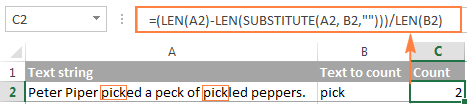#### Case-sensitive formula to count specific words in a cell

As you probably know, Excel SUBSTITUTE is a case-sensitive function, and therefore the word counting formula based on SUBSTITUTE is case-sensitive by default: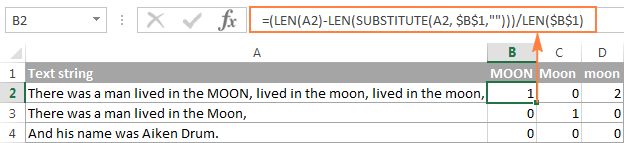### Case-insensitive formula to count specific words in a cell

If you need to count both uppercase and lowercase occurrences of a given word, use either the UPPER or LOWER function inside SUBSTITUTE to convert the original text and the text you want to count to the same case.

=(LEN(cell)-LEN(SUBSTITUTE(UPPER(cell),UPPER(text),"")))/LEN(text)

Or

=(LEN(cell)-LEN(SUBSTITUTE(LOWER(cell),LOWER(text),"")))/LEN(text)

For example, to count the number of occurrences of the word in B1 within cell A2 ignoring case, use this formula:

`=(LEN(A2)-LEN(SUBSTITUTE(LOWER(A2),LOWER(\$B\$1),"")))/LEN(\$B\$1)`

As demonstrated in the below screenshot, the formula returns the same word count regardless of whether the word is typed in UPPERCASE (cell B1), lowercase (cell D1) or Sentence case (cell C1):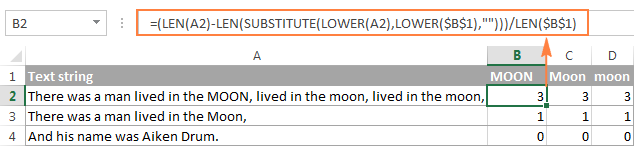## Count the total number of words in a range

To find out how many words a certain range contains, take the formula that counts total words in a cell and embed it within either SUMPRODUCT or SUM function:

=SUMPRODUCT(LEN(TRIM(range))-LEN(SUBSTITUTE(range," ",""))+1)

Or

=SUM(LEN(TRIM(range))-LEN(SUBSTITUTE(range," ",""))+1)

SUMPRODUCT is one of few Excel functions that can handle arrays, and you complete the formula in the usual way by pressing the Enter key.

For the SUM function to calculate arrays, it should be used in an array formula, which is completed by pressing Ctrl+Shift+Enter instead of the usual Enter stroke.

For example, to count all words in range A2:A4, use one of the following formulas:

`=SUMPRODUCT(LEN(TRIM(A2:A4))-LEN(SUBSTITUTE(A2:A4," ",""))+1)`

`=SUM(LEN(TRIM(A2:A4))-LEN(SUBSTITUTE(A2:A4," ",""))+1)`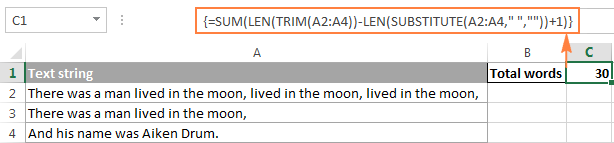## Count specific words in a range

If you want to count how many times a particular word or text appears within a range of cells, use a similar approach - take the formula to count specific words in a cell, and combine it with the SUM or SUMPRODUCT function:

=SUMPRODUCT((LEN(range)-LEN(SUBSTITUTE(range, word,"")))/LEN(word))

Or

=SUM((LEN(range)-LEN(SUBSTITUTE(range, word,"")))/LEN(word))

Please remember to press Ctrl+Shift+Enter to correctly complete the array SUM formula.

For example, to count all occurrences of the word entered in cell C1 within the range A2:A4, use this formula:

`=SUMPRODUCT((LEN(A2:A4)-LEN(SUBSTITUTE(A2:A4, C1,"")))/LEN(C1))`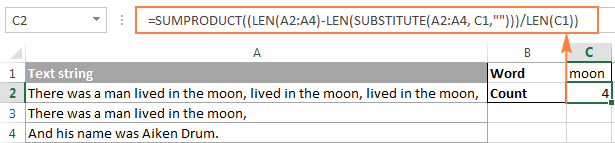As you remember, SUBSTITUTE is a case-sensitive function, and therefore the above formula distinguishes between uppercase and lowercase text: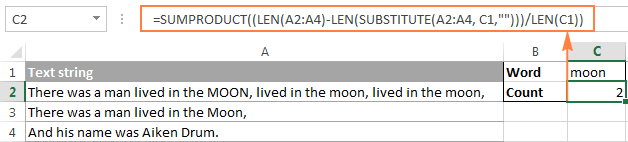To make the formula case-insensitive, use either the UPPER or LOWER function:

`=SUMPRODUCT((LEN(A2:A4)-LEN(SUBSTITUTE((UPPER(A2:A4)),UPPER(C1),"")))/LEN(C1))`

Or

`=SUMPRODUCT((LEN(A2:A4)-LEN(SUBSTITUTE((LOWER(A2:A4)),LOWER(C1),"")))/LEN(C1))`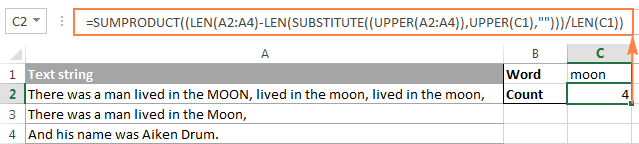This is how you count words in Excel. To better understand and probably reverse-engineer the formulas, you are welcome to download the sample Excel Count Words workbook.

If none of the formulas discussed in this tutorial has solved your task, please check out the following list of resources that demonstrate other solutions to count cells, text and individual characters in Excel.

### 24 responses to "How to count words in Excel"

1.Jenal says:

I Have been following this site for several month. I found it give me complete and deep explanation about some excel feature. Thanks

2.sally says:

Can someone show me the formula needed to count how many times certain text appears in one column (the text can appear multiple times in one cell or just once) while meeting criteria from another cell.

For Example:

YEAR ........ACTION TAKEN
2010 .......Case 1: No Action, Case 2: No Action
2015 .......Case 1: Suspension, Case 2: Termination
2016 .......Case 1: No Action
2012 .......Case 1: Letter, Case 2: Termination
2010 .......Case 1: Suspension, Case 2: No Action
2010 .......Case 1: Letter, Case 2: No Action, Case 3: No Action
2013 .......Case 1: Suspension

I need a formula that will count the number of times in 2010, No Action was taken in cases. The answer is 3.

This formula:

=SUM((LEN(range)-LEN(SUBSTITUTE(range, word,"")))/LEN(word))

Gets me halfway there. Now I need to figure out how to only count the words when the YEAR row is 2010.

3.rizwan says:

hi in excal i want to this date format 2016-06 to do less 1 after less 1 new value 2016-06 and if my value 2017-01 after calculate my new answer 2016-12 tell me how it possible this

4.Chris says:

Hello,
I am trying to find a method to use this formula:
=SUM((LEN(Page_1!H:H)-LEN(SUBSTITUTE((UPPER(Page_1!H:H)),UPPER(A3),"")))/LEN(A3))

And
Have it only calculate based on a date range from A:A (date/time formatted)

How can I do this?

5.Lauren says:

I need total word count in a cell, done line by line. I pasted this formula and nothing calculates, just the formula in the cell. Can someone help?

=LEN(TRIM(D3))-LEN(SUBSTITUTE(D3," ",""))+1

Thank you.

6.vikram says:

I need to compere the Characters & numbers with true or false

Reference Qty True/False

d1, d2, d3 3 TRUE
d1, d2, d4,d5 5 FALSE

7.vikram says:

Reference Qty True/False

d1, d2, d3 3 TRUE
d1, d2, d4,d5 5 FALSE

8.MEER SHUAIB says:

Hi,

Wanted to count of the words in huge excel sheet. Succeeded through this page.

Thanks for the help. Couldn't find else where in Internet.

9.Britt says:

Thank you so much! I was trying to use "split" with array formula and it wasn't working, but your first example with the len(trim( worked very well with arrayformula and saved my project :).

10.Sepideh Asadi says:

An easier solution with the following builtin formula in Excel:
COUNTIF(range,criteria)

eg: (B1:B10 , "Yes")

Counts the number of cells within a range that meet the given condition, here "Yes".

▪ range: is the range of cells from which you want to count nonblank cells.
▪ criteria: is the condition in the form of a number, expression, or text that defines which cells will be counted.

•Sepideh Asadi says:

Sorry this reply is posted by mistake. I have no clue how can I delete it.

11.gary says:

Nice. thank you.

12.Andi says:

This was very helpful! Thank you!
Actually in the formula instead of commas, there should be semicolon:

=LEN(TRIM(A1))-LEN(SUBSTITUTE(A1; " "; ""))+1

•Doug says:

Andi:
The semicolon vs comma is a regional setting in Office. The formulas will provide the result desired.

13.Giselle says:

Hi I am trying to count the words in a cell after a specific character. I am having trouble nesting the subtitue function to count the words into the character count after ")".

I have this so far:
LEN(Right(AP2;LEN(AP2)-SEARCH(")");AP2)
LEN(SUBSTITUTE(AP2;" ";""))+1

How can I combine those two?

Thanks a lot!!

•Giselle says:

I just saw a mistake in the first LEN function. I removed the second bracket in the SEARCH Function. But still would need help in nesting the Substitute function!

Thanks a lot!!

•Svetlana Cheusheva (Ablebits.com Team) says:

Hi Giselle,

Possibly, there is a more compact version of the below formula, but it is the only one I've managed to get to work :)

=IFERROR(LEN(TRIM(RIGHT(AP2;LEN(AP2)-SEARCH(")"; AP2)-1)))-LEN(SUBSTITUTE(RIGHT(AP2;LEN(AP2)-SEARCH(")"; AP2)-1);" ";""))+1; 0)

14.Ricardo says:

How can count number of characters before the first space, for example in the cell the text is: "Aspirin is a pill" the result should be 7

Thank you

•Mary Trifuntova (Ablebits.com Team) says:

Hello, Ricardo,

=LEN(LEFT(A1, SEARCH(" ", A1)-1))

Where A1 is the cell that contains your text string. After that, you can copy this formula down along the column if necessary.
Hope this is what you need.

15.vamsi emani says:

Hi all,
I would really appreciate if someone could help me with this issue.
I recently figured out how to select multiple options in a drop down menu. Example say loader, truck, compressor, bus are the options. Selecting these options at once without repetitions gave me the addition of the costs using the formula below

A B
1. Bus, Truck, Compressor, Loader 1380\$
2. Bus, Truck 410\$
3. Bus, Truck, Truck 410\$

'formula on B Column'=SUMPRODUCT(--(ISNUMBER(SEARCH(\$D\$1:\$D\$4,A1))),\$E\$1:\$E\$4)

D E
Equipment Cost
2. Truck 150\$
3. Compressor 120\$
4. Bus 260\$

Now, I need a formula that could give result even if i select options repetitively. Example, selecting truck twice in the above option did not add up to the final number. ( Note: i am able to select more than once on the drop down menu, only issue is its not adding up).

16.vamsi emani says:

Hi all,
I would really appreciate if someone could help me with this issue.
I recently figured out how to select multiple options in a drop down menu. Example say loader, truck, compressor, bus are the options. Selecting these options at once without repetitions gave me the addition of the costs using the formula below

___________A______________________________ B
1. Bus, Truck, Compressor, Loader ______1380\$
2. Bus, Truck____________________________410\$
3. Bus, Truck, Truck ____________________410\$

'formula on B Column'=SUMPRODUCT(--(ISNUMBER(SEARCH(\$D\$1:\$D\$4,A1))),\$E\$1:\$E\$4)

__D__________________E
Equipment__________Cost
2. Truck __________150\$
3. Compressor______120\$
4. Bus ____________260\$

Now, I need a formula that could give result even if i select options repetitively. Example, selecting truck twice in the above option did not add up to the final number. ( Note: i am able to select more than once on the drop down menu, only issue is its not adding up).

17.Sandaka Madhu Babu says:
18.Hassan says: